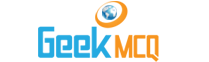Verbal and Reasoning :: Quantitative Comparison

1.  If the average of 5 and K is equal to the average of 2 6 and K. what is the value of K?
 A. 2 B. 8 C. 1 D. 3 E. 4

2.  How much the speed of a train be increased if the dirver wishes to reduce the time to reach to a certain station in 20% less time?
 A. 10% B. 25% C. 5% D. 20% E. 50%

3.  What is the probability of not coming a number greater than 2 when a die is thrown?
 A. 0.1 B. 0.2 C. 0.3 D. 0.4 E. 0.5

4.  If 4 men or 7 boys can do a work in 29 days then 12 men and 8 boys will do the same work in:
 A. 29 B. 17 C. 7 D. 21 E. 30

5.  A man spent 10% of his money. After spending 60% of the remainder he has Rs. 72 left. How much had he in the start?
 A. 300 B. 50 C. 400 D. 200 E. 100

6.  What is the largest of 13 consecutive integers whose sum is 0?
 A. -13 B. -6 C. 6 D. 9 E. 13

7.  A boy scored 90 marks for his mathematics test. This was 20% more than what he had scored for the geography test. How much did he score in geography
 A. 100 B. 75 C. 50 D. 180 E. 72

8.  What is the Next number in the geometric progression 4 12 36?
 A. 200 B. 172 C. 480 D. 108 E. 218

9.  The average (arithmetic mean) of five numbers is 26. After one of the numbers is removed the average (arithmetic mean) of the remaining number is 25. What number has been removed?
 A. 20 B. 25 C. 26 D. 30 E. 32

10.  If a = 3 then what is the value of (a2)3 - a?
 A. 12 B. 15 C. 240 D. 243 E. 726